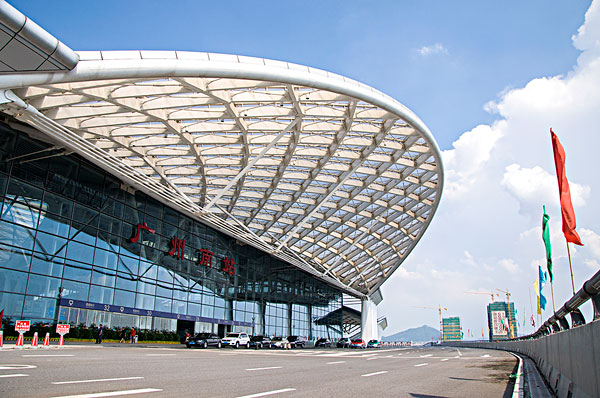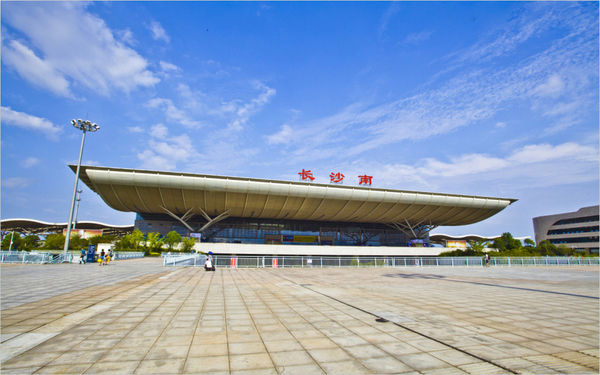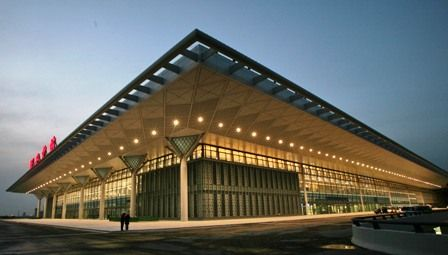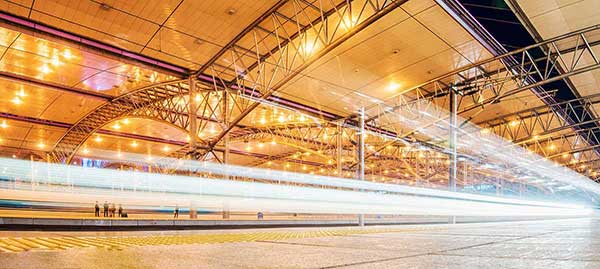•廣州南站

可用休息室6個

可用餐廳5個

•深圳北站

可用休息室5個

可用餐廳0個

•長沙南站

可用休息室4個

可用餐廳1個

•重慶北站

可用休息室3個

可用餐廳0個

•西安北站

可用休息室3個

可用餐廳2個

•瀋陽北站

可用休息室1個

可用餐廳0個

• 高鐵列表
• 全部地點 中國大陸 其他地區

A

• 奥伦堡火车站俄罗斯联邦

B

• 北京西站

• 璧山站

• 北京站中国

• 北京南站

C

• 長春西站

• 成都東站

• 長沙南站

HOT
• 重慶北站

HOT
• 车里雅宾斯火车站俄罗斯联邦

D

• 大足南站

• 大連北站

• 大連站

• 垫江站中国

F

• 福州站中国

• 福岡高鐵博多站日本

G

• 廣州南站

HOT
• 贵阳北站中国

• 桂林北站中国

H

• 合肥南站

• 弘大合井站韓國

• 杭州站中国

• 漢口站中国

• 杭州東站

• 哈爾濱西站

J

• 濟南西站

• 簡陽站

K

• 京都站日本

• 昆明南站中国

L

• 羅馬特米尼火車站義大利

• 隆昌北站

• 蘭州西站

• 梁平南站中国

• 柳州站中国

M

• 明洞站韓國

N

• 南寧東站中国

• 南昌西站

• 南寧站

• 內江北站

• 南京南站

• 南昌火車站

• 寧波站

O

• 大阪高鐵站日本

Q

• 秋明火车站俄罗斯联邦

• 青島北站

• 泉州站中国

R

• 榮昌北站

S

• 蘇州站

• 蘇州北站

• 石家莊站

• 瀋陽北站

HOT
• 三亞站

• 上海站

• 上海虹橋站

• 首爾站韓國

• 深圳北站

HOT

T

• 太原南站

• 太原站

• 天津南站

• 天津西站

W

• 無錫站

• 無錫東站

• 万州北站中国

• 無錫新區站

• 溫州南站

• 武漢站

• 乌兰乌德火车站俄罗斯联邦

X

• 徐州東站

• 廈門北站

• 西安北站

HOT
• 新西伯利亚火车站俄罗斯联邦

Y

• 伊尔库茨克火车站俄罗斯联邦

• 永川東站

• 叶卡捷琳堡火车站俄罗斯联邦

Z

• 中山站

• 資陽北站

• 鄭州東站

• 資中北站

• 珠海站

A

B

C

D

F

G

H

J

K

L

M

N

O

Q

R

S

T

W

X

Y

Z